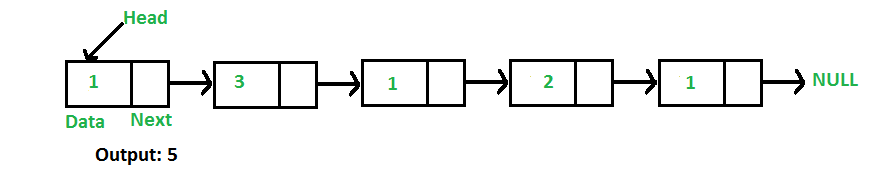# Javascript Program For Finding Length Of A Linked List

• Last Updated : 16 Jun, 2022

Write a function to count the number of nodes in a given singly linked list.For example, the function should return 5 for linked list 1->3->1->2->1.

Iterative Solution:

```1) Initialize count as 0
2) Initialize a node pointer, current = head.
3) Do following while current is not NULL
a) current = current -> next
b) count++;
4) Return count ```

Following is the Iterative implementation of the above algorithm to find the count of nodes in a given singly linked list.

## Javascript

 ``

Output:

`count of nodes is 5`

Time Complexity: O(n), where n represents the length of the given linked list.
Auxiliary Space: O(1), no extra space is required, so it is a constant.

Recursive Solution:

```int getCount(head)
1) If head is NULL, return 0.
2) Else return 1 + getCount(head->next) ```

Following is the Recursive implementation of the above algorithm to find the count of nodes in a given singly linked list.

## Javascript

 ``

Output:

`Count of nodes is 5`

Time Complexity: O(n), where n represents the length of the given linked list.
Auxiliary Space: O(n), for recursive stack where n represents the length of the given linked list.

Please refer complete article on Find Length of a Linked List (Iterative and Recursive) for more details!

My Personal Notes arrow_drop_up